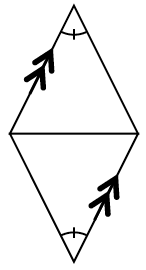### Home > GC > Chapter 8 > Lesson 8.1.5 > Problem8-54

8-54.

Multiple Choice: The triangles below are congruent because of:

1. SSA $\cong$

1. HL $\cong$

1. SAS $\cong$

1. SSS $\cong$

1. none of these

What special types of angles are present in this triangle? Think parallel lines.
Are there any sides that are congruent?
Do you have enough information to say the triangles are congruent?
Which conjecture matches the information you determined?